# Trigonometric Functions The Unit Circle Section 4 2

• Slides: 67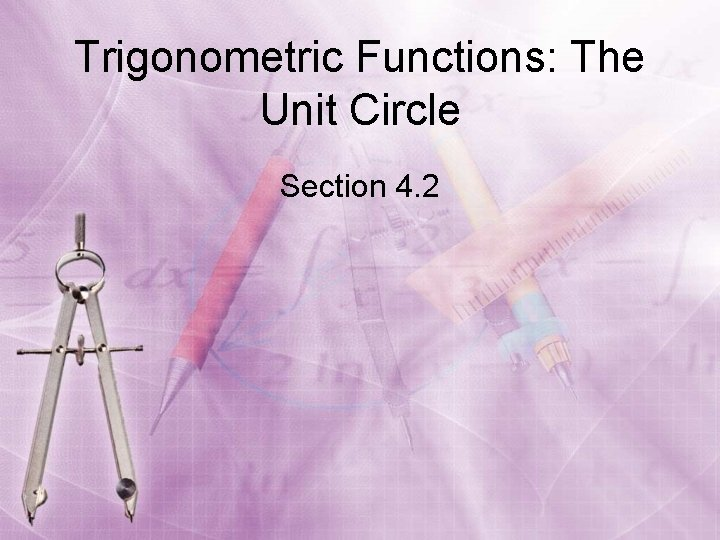Trigonometric Functions: The Unit Circle Section 4. 2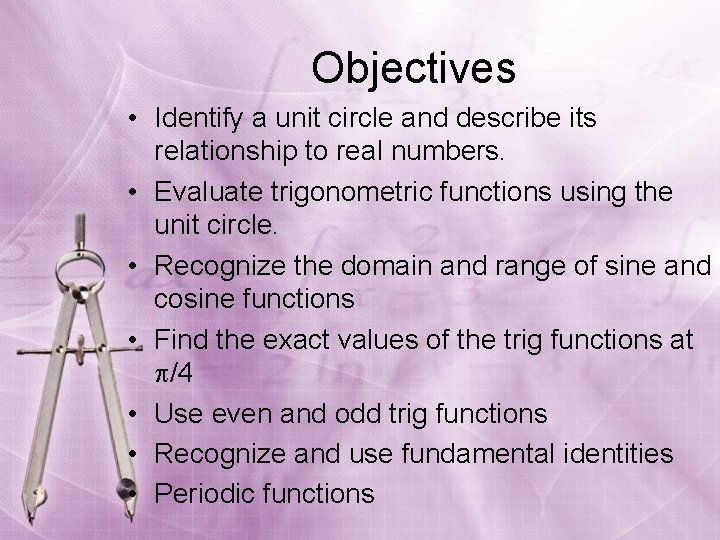Objectives • Identify a unit circle and describe its relationship to real numbers. • Evaluate trigonometric functions using the unit circle. • Recognize the domain and range of sine and cosine functions • Find the exact values of the trig functions at /4 • Use even and odd trig functions • Recognize and use fundamental identities • Periodic functions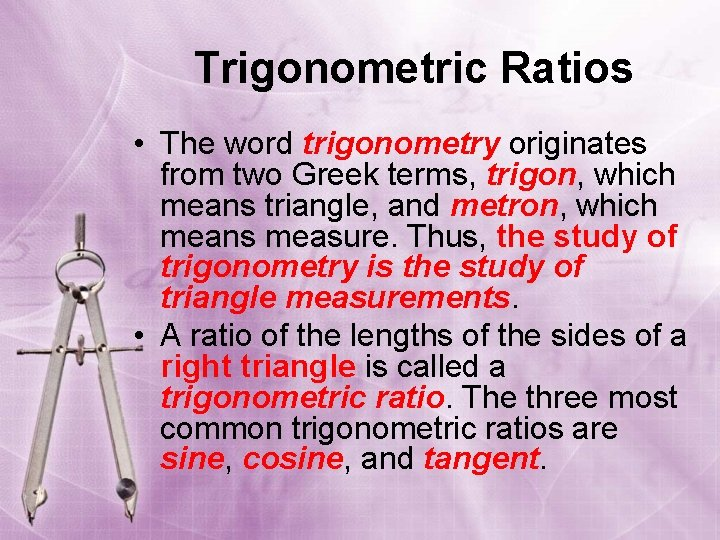Trigonometric Ratios • The word trigonometry originates from two Greek terms, trigon, which means triangle, and metron, which means measure. Thus, the study of trigonometry is the study of triangle measurements. • A ratio of the lengths of the sides of a right triangle is called a trigonometric ratio. The three most common trigonometric ratios are sine, cosine, and tangent.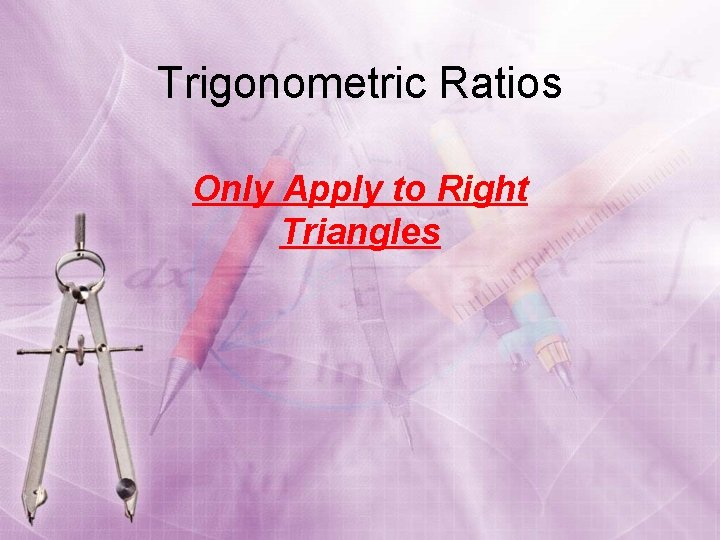Trigonometric Ratios Only Apply to Right Triangles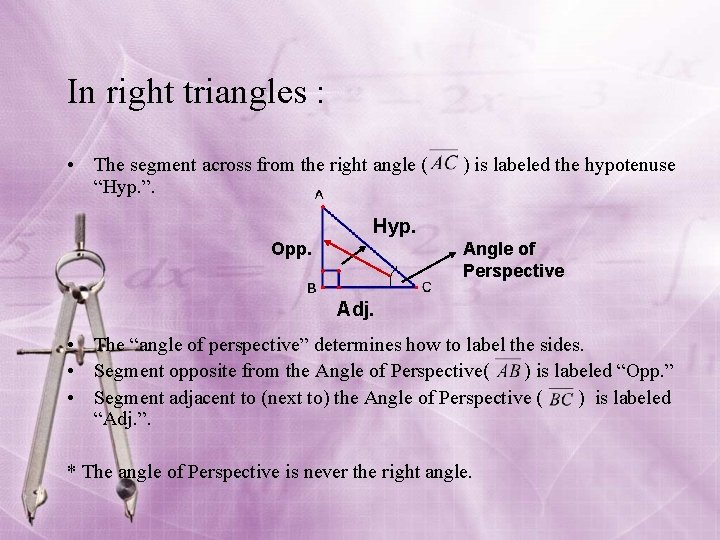In right triangles : • The segment across from the right angle ( ) is labeled the hypotenuse “Hyp. ”. Hyp. Opp. Angle of Perspective Adj. • The “angle of perspective” determines how to label the sides. • Segment opposite from the Angle of Perspective( ) is labeled “Opp. ” • Segment adjacent to (next to) the Angle of Perspective ( ) is labeled “Adj. ”. * The angle of Perspective is never the right angle.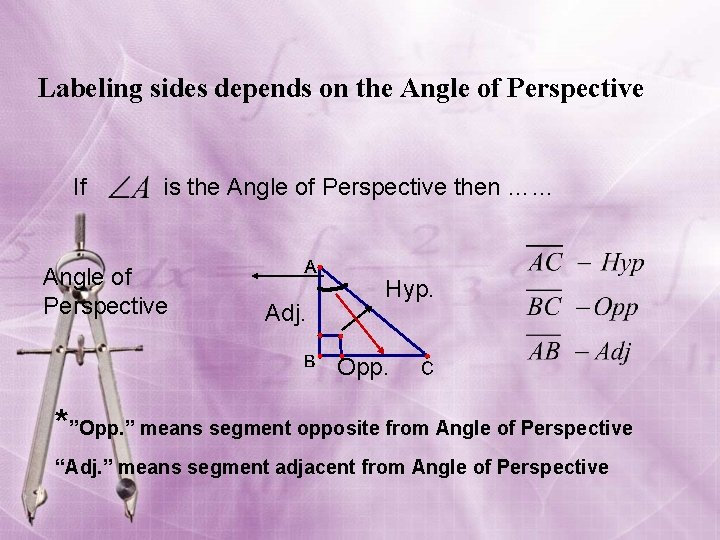Labeling sides depends on the Angle of Perspective If is the Angle of Perspective then …… Angle of Perspective Adj. Hyp. Opp. *”Opp. ” means segment opposite from Angle of Perspective “Adj. ” means segment adjacent from Angle of Perspective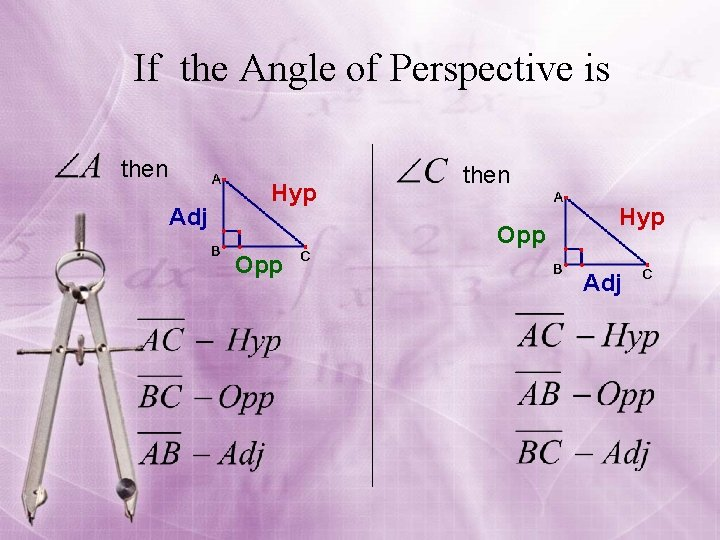If the Angle of Perspective is then Adj Hyp then Opp Hyp Adj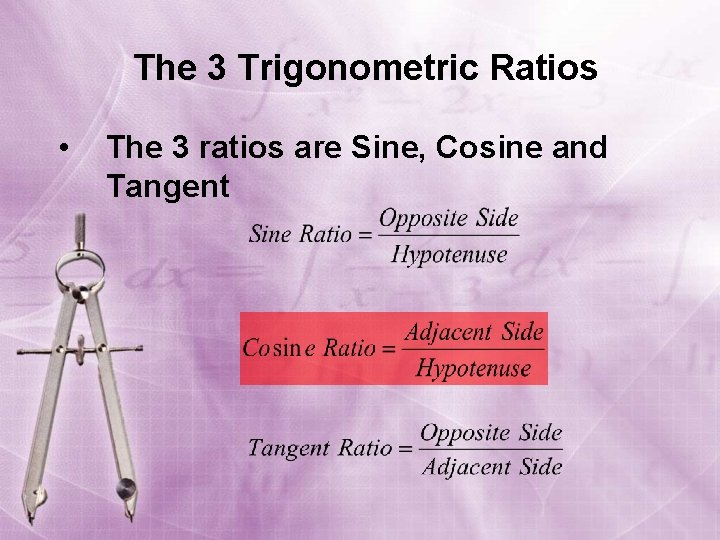The 3 Trigonometric Ratios • The 3 ratios are Sine, Cosine and TangentThe Amazing Legend of… Chief Soh. Cah. ToaChief Soh. Cah. Toa • Once upon a time there was a wise old Native American Chief named Chief Soh. Cah. Toa. • He was named that due to an chance encounter with his coffee table in the middle of the night. • He woke up hungry, got up and headed to the kitchen to get a snack. • He did not turn on the light and in the darkness, stubbed his big toe on his coffee table…. Please share this story with Mr. Gustin for historical credibility.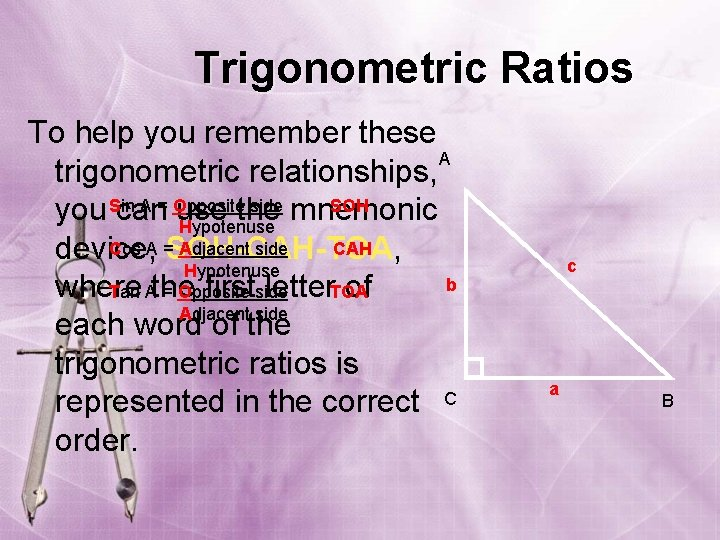Trigonometric Ratios To help you remember these A trigonometric relationships, Sin A = Opposite side SOH you can use the mnemonic Hypotenuse Cos A = Adjacent side CAH device, SOH-CAH-TOA, Hypotenuse b where the first letter of Tan A = Opposite side TOA Adjacent side each word of the trigonometric ratios is represented in the correct C order. c a B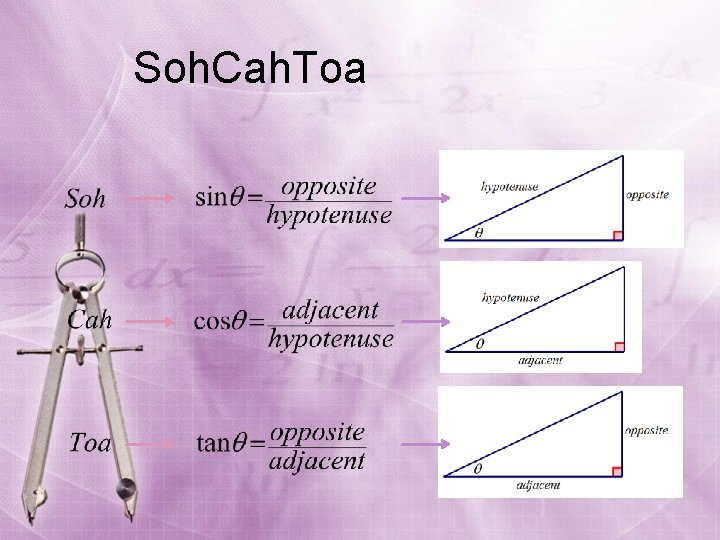Soh. Cah. ToaThe six trigonometric functions of a right triangle, with an acute angle , are defined by ratios of two sides of the triangle. The sides of the right triangle are: hyp the side opposite the acute angle , the side adjacent to the acute angle θ , and the hypotenuse of the right triangle. adj The trigonometric functions are sine, cosine, tangent, cotangent, secant, and cosecant. opp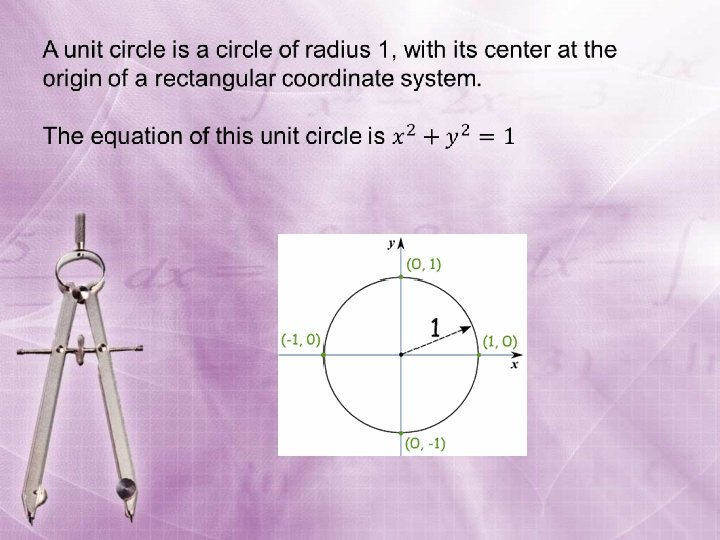The Unit Circle • Here we have a unit circle on the coordinate plane, with its center at the origin, and a radius of 1. • The point on the circle is in quadrant I.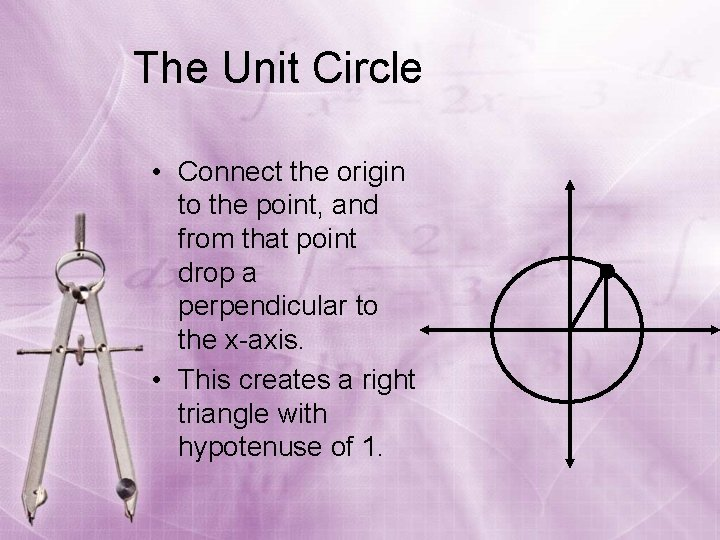The Unit Circle • Connect the origin to the point, and from that point drop a perpendicular to the x-axis. • This creates a right triangle with hypotenuse of 1.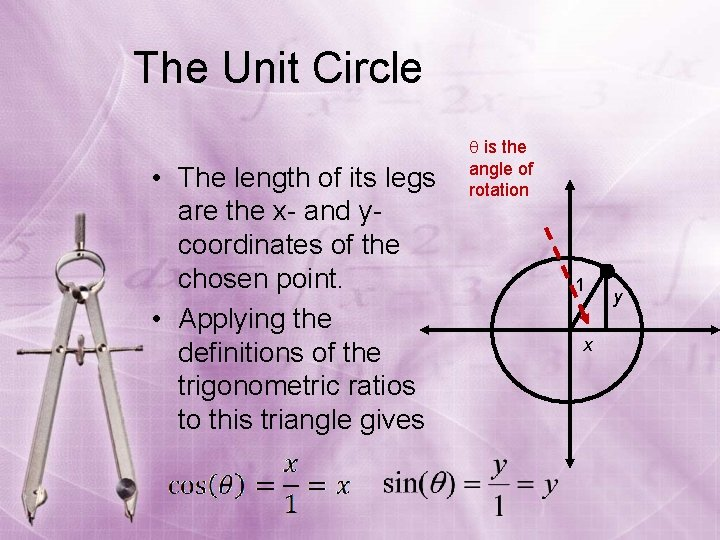The Unit Circle • The length of its legs are the x- and ycoordinates of the chosen point. • Applying the definitions of the trigonometric ratios to this triangle gives is the angle of rotation 1 x yThe Unit Circle • The coordinates of the chosen point are the cosine and sine of the angle . – This provides a way to define functions sin( ) and cos( ) for all real numbers . – The other trigonometric functions can be defined from these.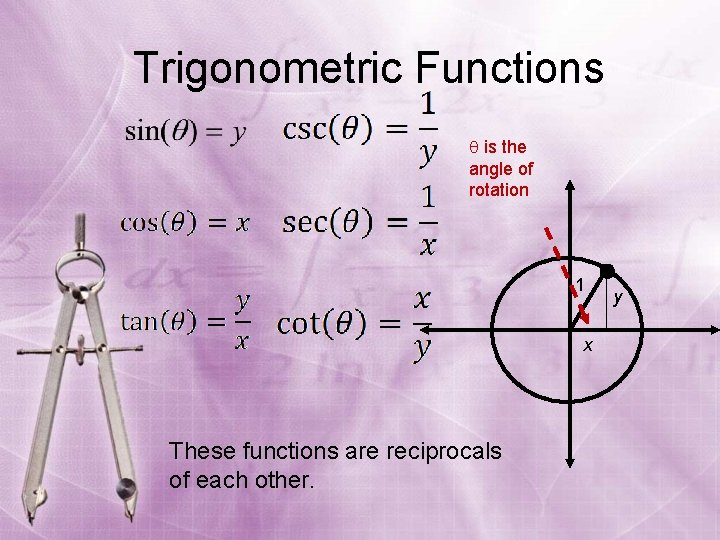Trigonometric Functions is the angle of rotation 1 x These functions are reciprocals of each other. y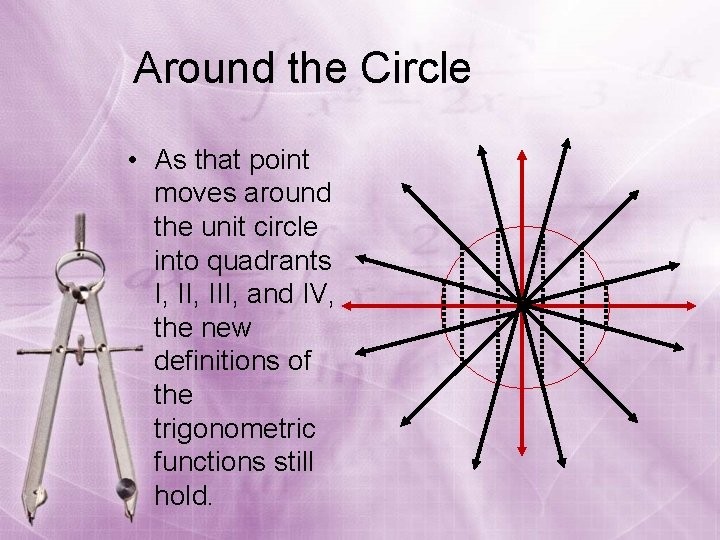Around the Circle • As that point moves around the unit circle into quadrants I, III, and IV, the new definitions of the trigonometric functions still hold.The Unit Circle sin One of the most useful tools in trigonometry is the unit circle. It is a circle, with radius 1 unit, that is on the x-y coordinate plane. 1 cos The x-axis corresponds to the cosine function, and the y-axis corresponds to the sine function. The angles are measured from the positive x-axis (standard position) counterclockwise. In order to create the unit circle, we must use the special right triangles below: 45º 1 1 60º 30º -60º -90º The hypotenuse for each triangle is 1 unit. 45º -45º -90º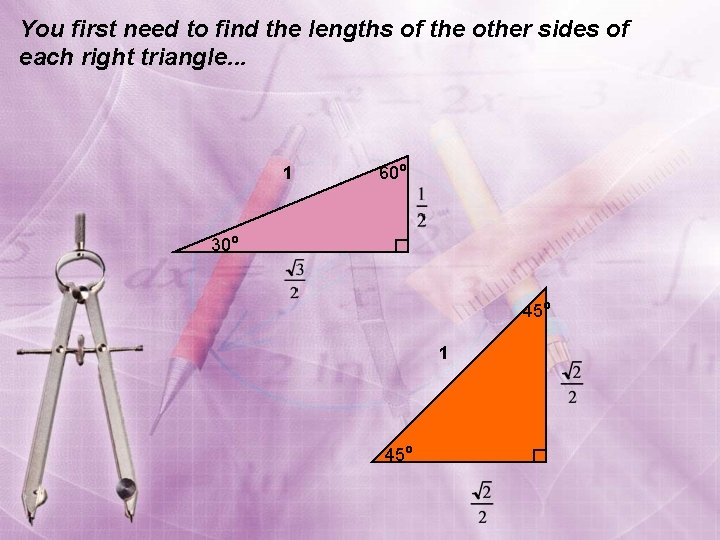You first need to find the lengths of the other sides of each right triangle. . . 1 60º 30º 45º 1 45ºUsefulness of Knowing Trigonometric Functions of Special Angles: 30 o, 45 o, 60 o • The trigonometric function values derived from knowing the side ratios of the 30 -60 -90 and 45 -4590 triangles are “exact” numbers, not decimal approximations as could be obtained from using a calculator • You will often be asked to find exact trig function values for angles other than 30 o, 45 o and 60 o angles that are somehow related to trig function values of these anglesNow, use the corresponding triangle to find the coordinates on the unit (0, 1) sin circle. . . What are the coordinates of This cooresponds this point? to (cos 30, sin 30) (Use your (cos 30, sin 30) 30 -60 -90 triangle) (– 1, 0) 30º cos (1, 0) (0, – 1)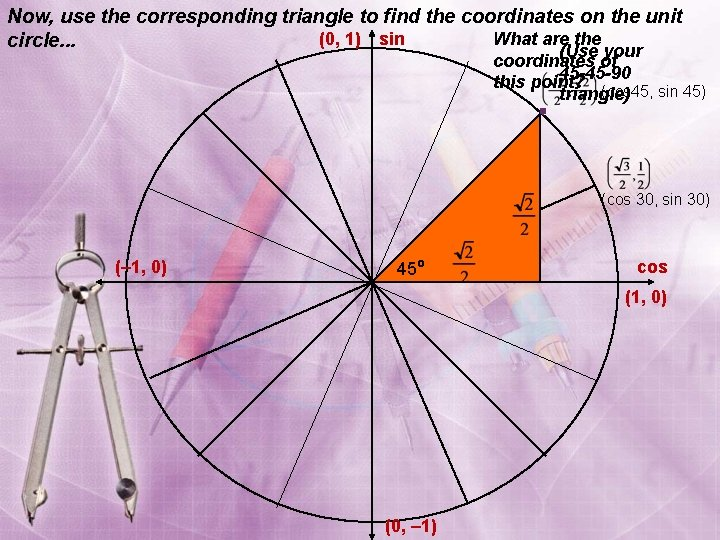Now, use the corresponding triangle to find the coordinates on the unit What are the (0, 1) sin circle. . . (Use your coordinates of 45 -45 -90 this point? (cos 45, sin 45) triangle) (cos 30, sin 30) (– 1, 0) 45º cos (1, 0) (0, – 1)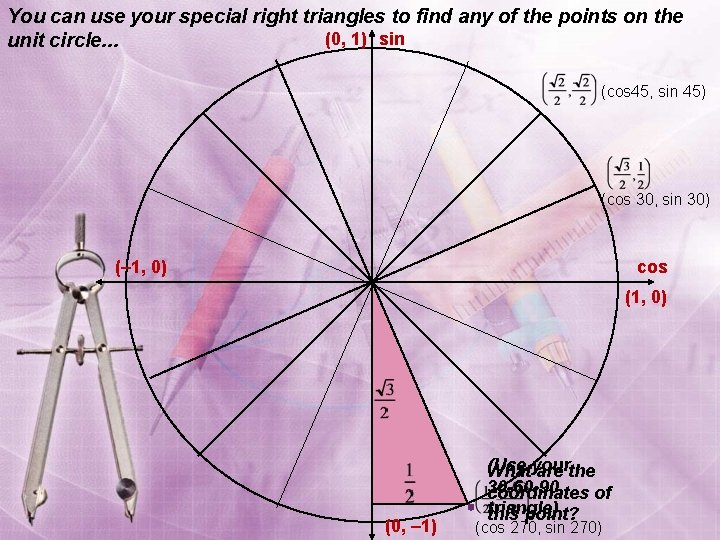You can use your special right triangles to find any of the points on the (0, 1) sin unit circle. . . (cos 45, sin 45) (cos 30, sin 30) (– 1, 0) cos (1, 0) (0, – 1) (Use Whatyour are the 30 -60 -90 coordinates of triangle) this point? (cos 270, sin 270)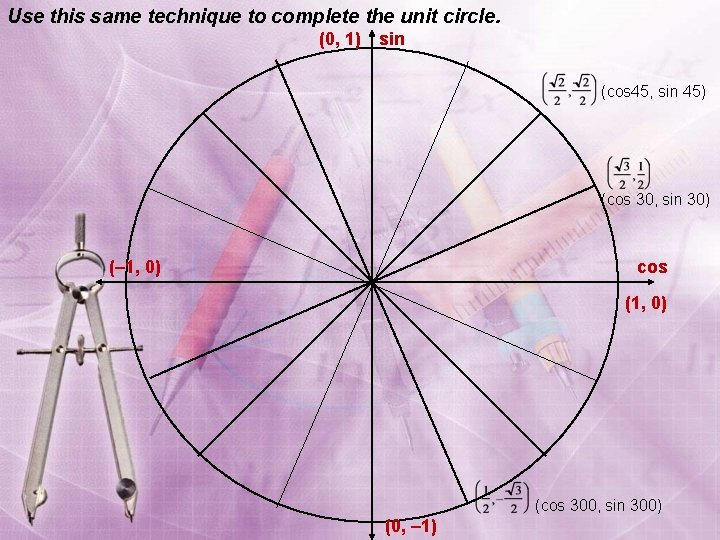Use this same technique to complete the unit circle. (0, 1) sin (cos 45, sin 45) (cos 30, sin 30) (– 1, 0) cos (1, 0) (cos 300, sin 300) (0, – 1)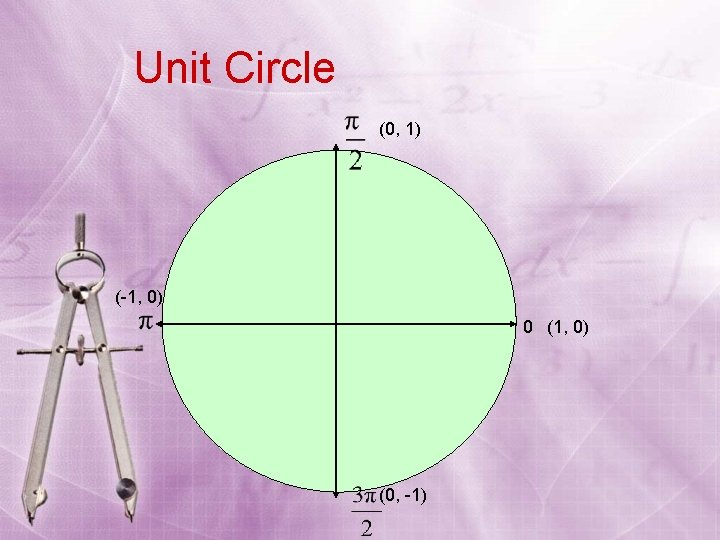Unit Circle (0, 1) (-1, 0) 0 (1, 0) (0, -1)Unit Circle (0, 1) 30° (-1, 0) 30° (1, 0) 30° (0, -1)Unit Circle (0, 1) (-1, 0) 60° 60° (0, -1)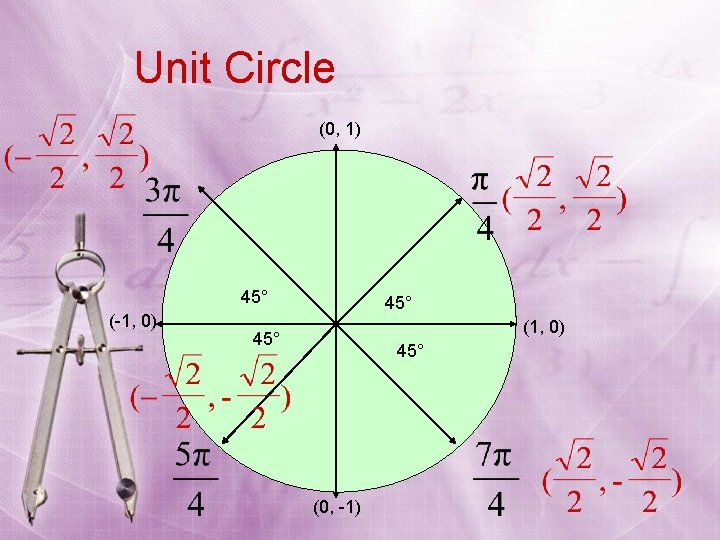Unit Circle (0, 1) 45° (-1, 0) 45° (1, 0) 45° (0, -1)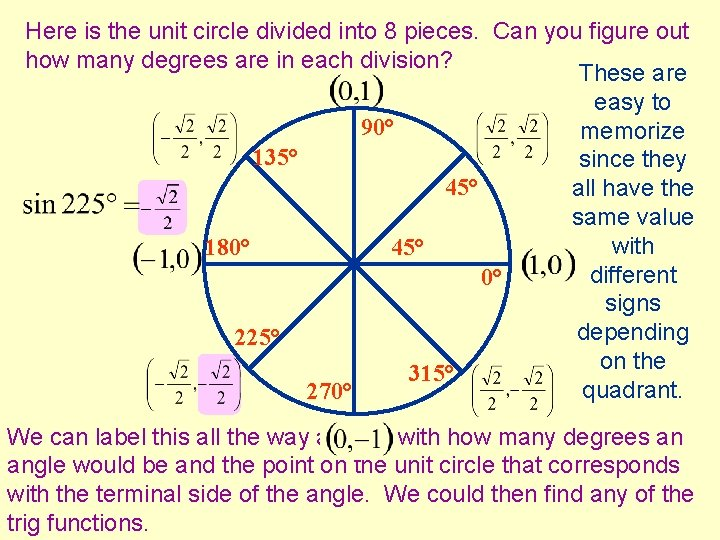Here is the unit circle divided into 8 pieces. Can you figure out how many degrees are in each division? These are easy to 90° memorize 135° since they 45° all have the same value with 180° 45° different 0° signs depending 225° on the 315° quadrant. 270° We can label this all the way around with how many degrees an angle would be and the point on the unit circle that corresponds with the terminal side of the angle. We could then find any of the trig functions.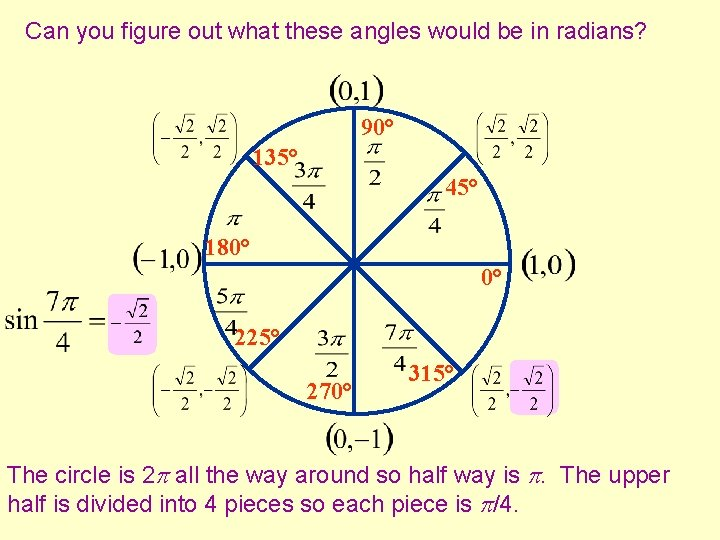Can you figure out what these angles would be in radians? 90° 135° 45° 180° 0° 225° 270° 315° The circle is 2 all the way around so half way is . The upper half is divided into 4 pieces so each piece is /4.Here is the unit circle divided into 12 pieces. Can you figure out how many degrees are in each division? You'll need to memorize 120° 90° 60° these too 150° but you can 30° see the pattern. 180° 30° 0° 210° 330° 240° 270° 300° We can again label the points on the circle and the sine is the y value, the cosine is the x value and the tangent is y over x.Can you figure out what the angles would be in radians? 120° 90° 60° 150° 30° 180° 30° 0° 210° 330° 240° 270° We'll see them all put together on the unit circle on the next screen. 300° It is still halfway around the circle and the upper half is divided into 6 pieces so each piece is /6.You should memorize this. This is a great reference because you can figure out the trig functions of all these angles quickly.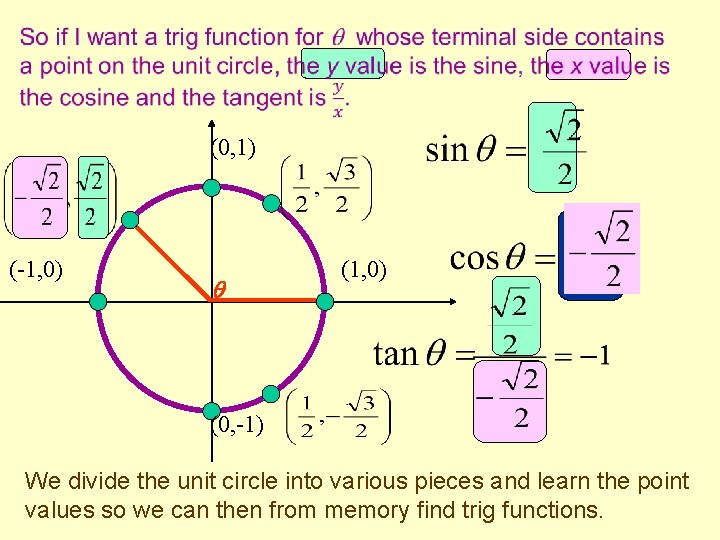(0, 1) (-1, 0) (0, -1) We divide the unit circle into various pieces and learn the point values so we can then from memory find trig functions.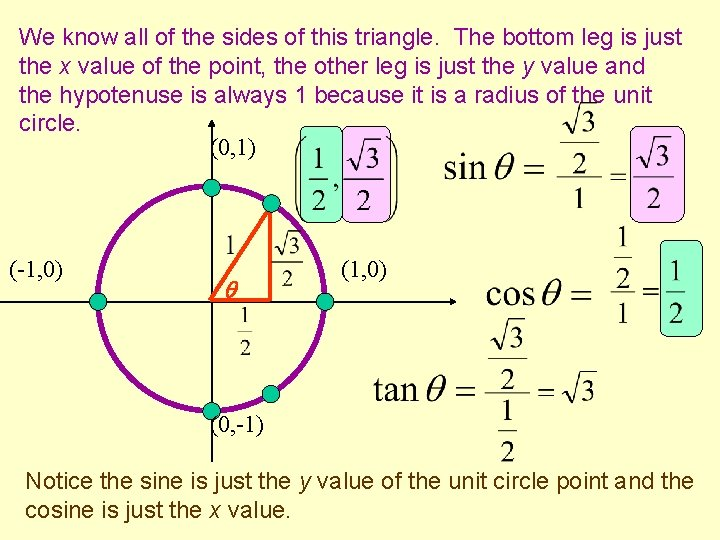We know all of the sides of this triangle. The bottom leg is just the x value of the point, the other leg is just the y value and the hypotenuse is always 1 because it is a radius of the unit circle. (0, 1) (-1, 0) (0, -1) Notice the sine is just the y value of the unit circle point and the cosine is just the x value.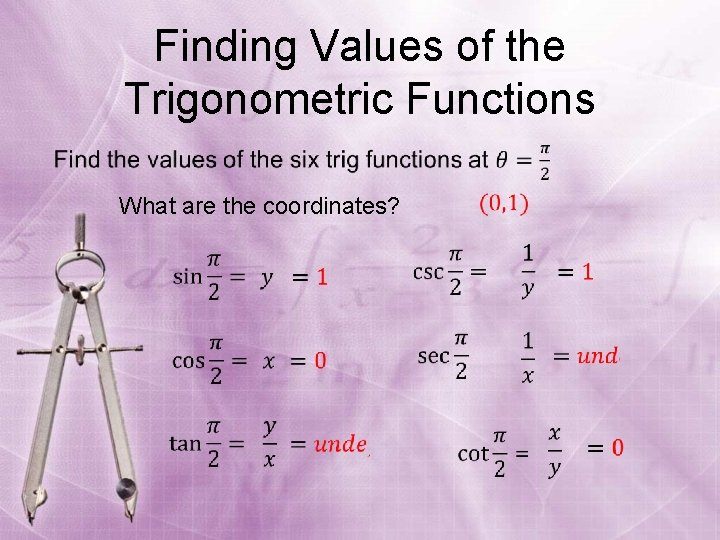Finding Values of the Trigonometric Functions What are the coordinates?Finding Values of the Trigonometric Functions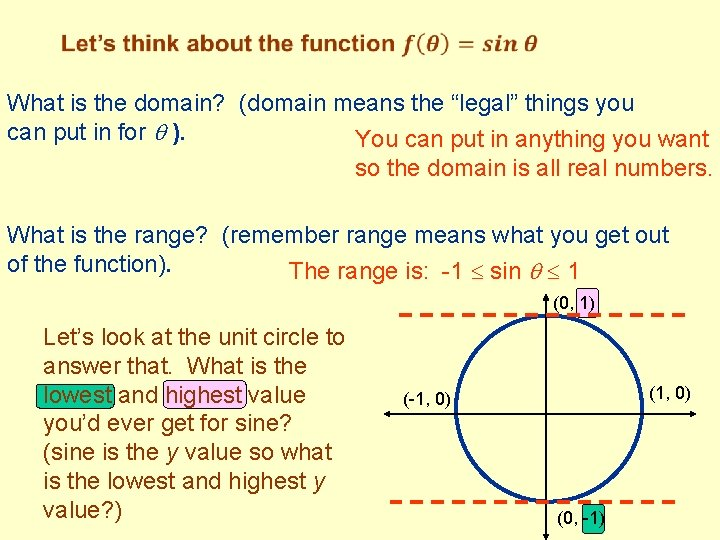What is the domain? (domain means the “legal” things you can put in for ). You can put in anything you want so the domain is all real numbers. What is the range? (remember range means what you get out of the function). The range is: -1 sin 1 (0, 1) Let’s look at the unit circle to answer that. What is the lowest and highest value you’d ever get for sine? (sine is the y value so what is the lowest and highest y value? ) (1, 0) (-1, 0) (0, -1)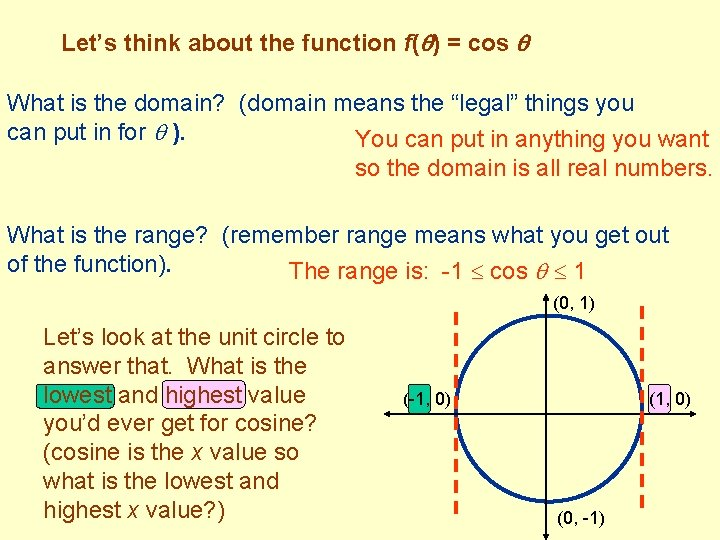Let’s think about the function f( ) = cos What is the domain? (domain means the “legal” things you can put in for ). You can put in anything you want so the domain is all real numbers. What is the range? (remember range means what you get out of the function). The range is: -1 cos 1 (0, 1) Let’s look at the unit circle to answer that. What is the lowest and highest value you’d ever get for cosine? (cosine is the x value so what is the lowest and highest x value? ) (-1, 0) (0, -1)Even and Odd Trig Functions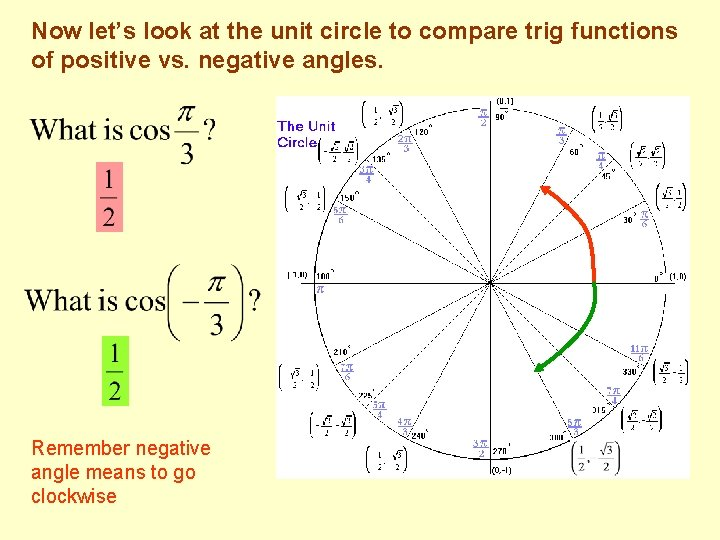Now let’s look at the unit circle to compare trig functions of positive vs. negative angles. Remember negative angle means to go clockwise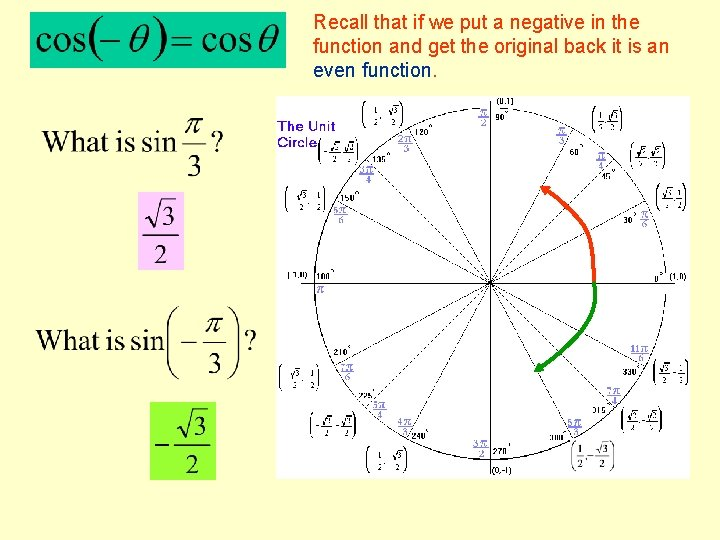Recall that if we put a negative in the function and get the original back it is an even function.Recall that if we put a negative in the function and get the negative of the function back it is an odd function.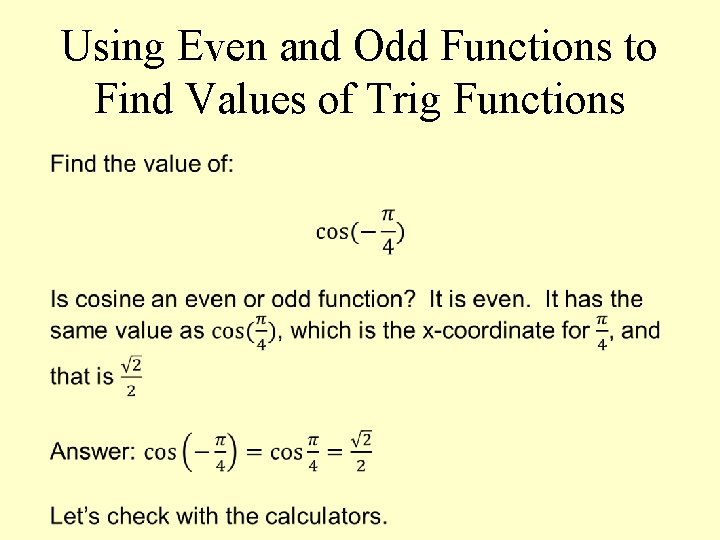Using Even and Odd Functions to Find Values of Trig Functions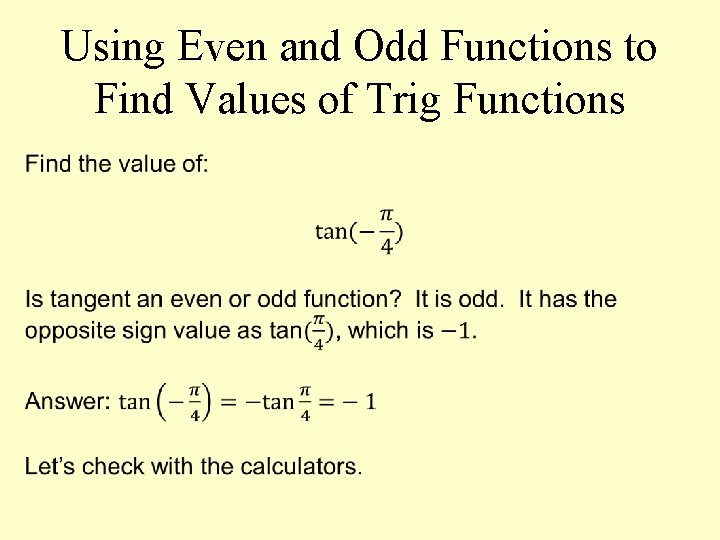Using Even and Odd Functions to Find Values of Trig Functions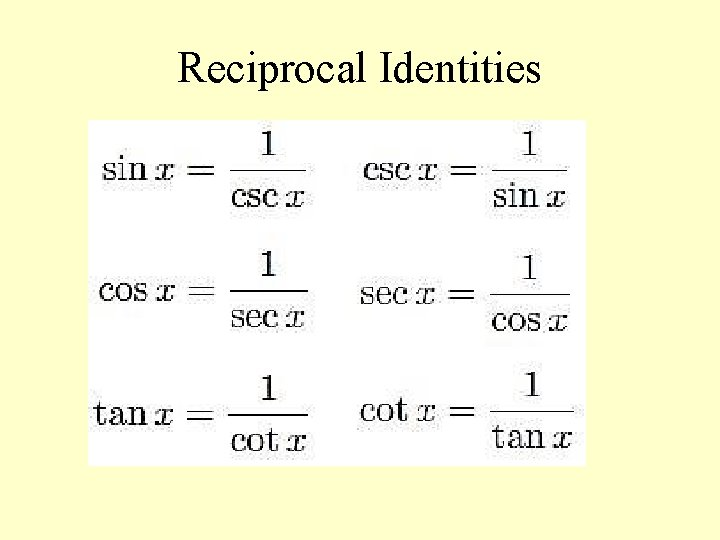Reciprocal IdentitiesQuotient Identities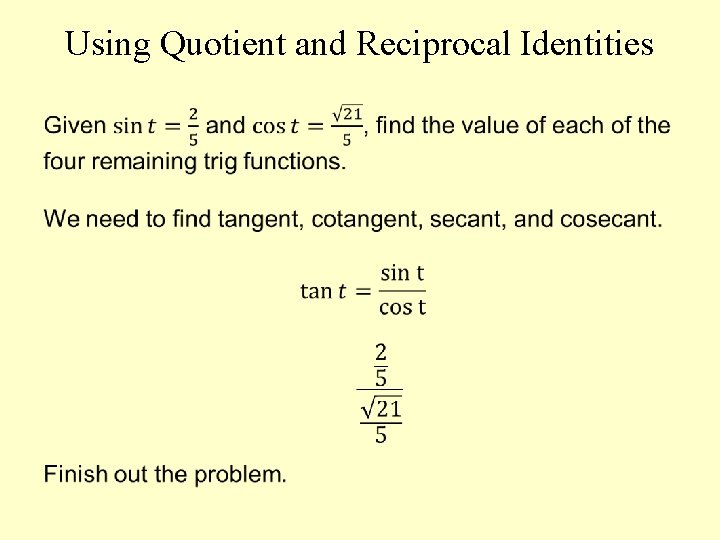Using Quotient and Reciprocal IdentitiesUsing Quotient and Reciprocal IdentitiesUsing Quotient and Reciprocal Identities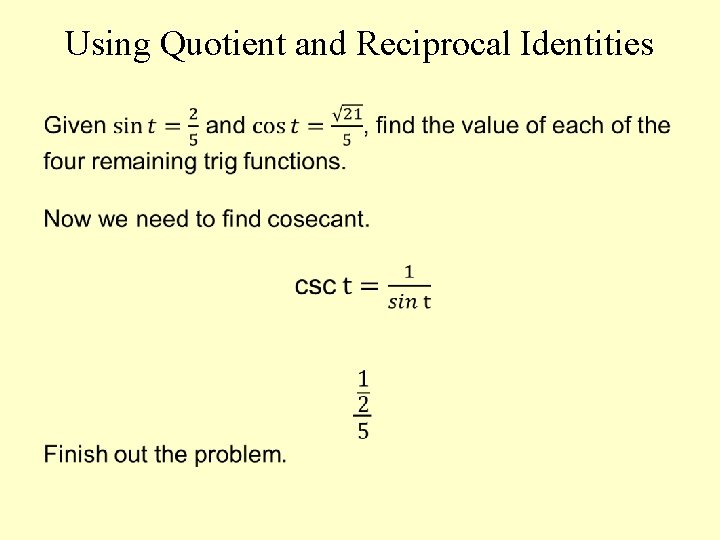Using Quotient and Reciprocal IdentitiesPythagorean Identities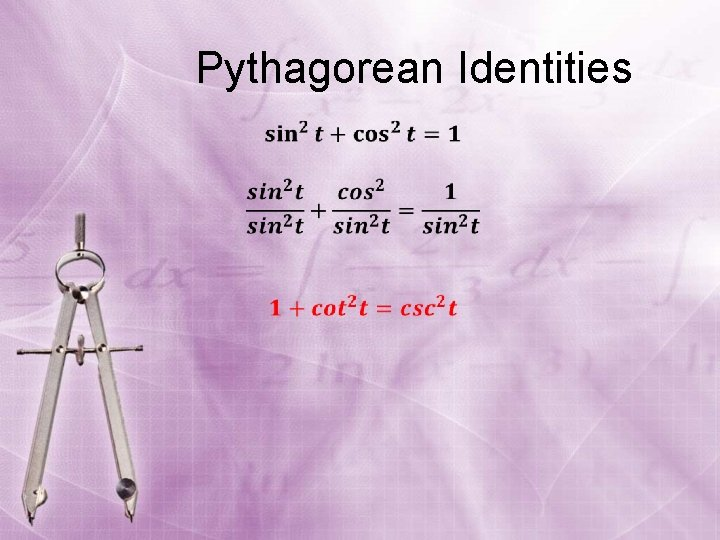Pythagorean Identities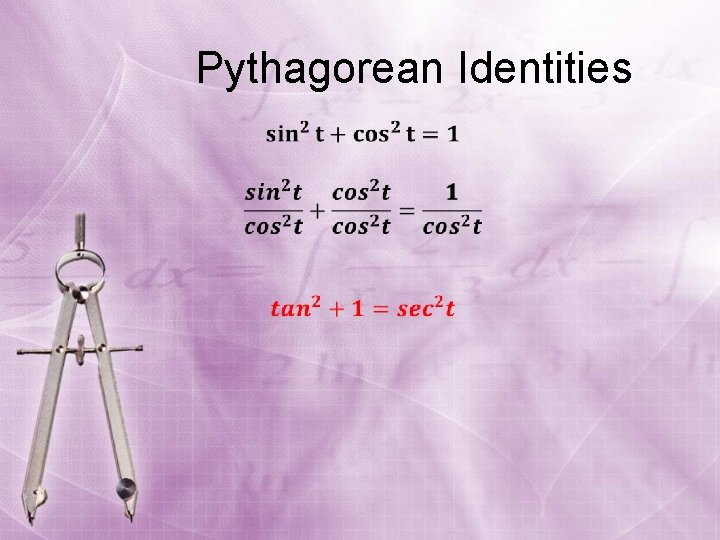Pythagorean IdentitiesUsing a Pythagorean Identity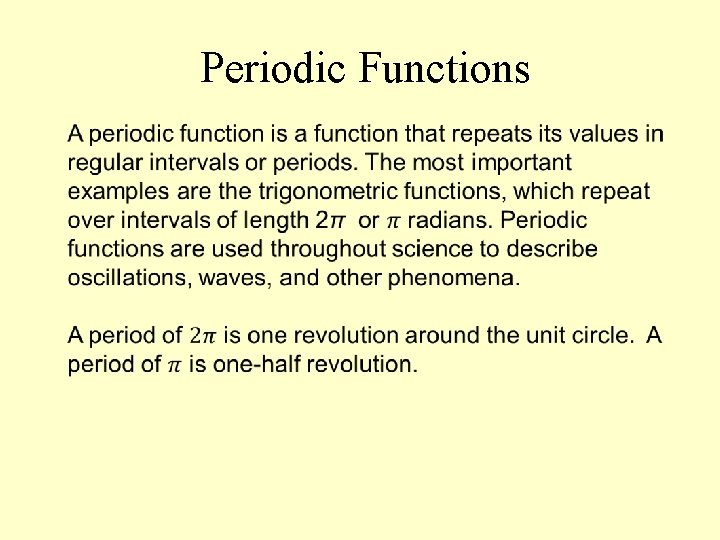Periodic Functions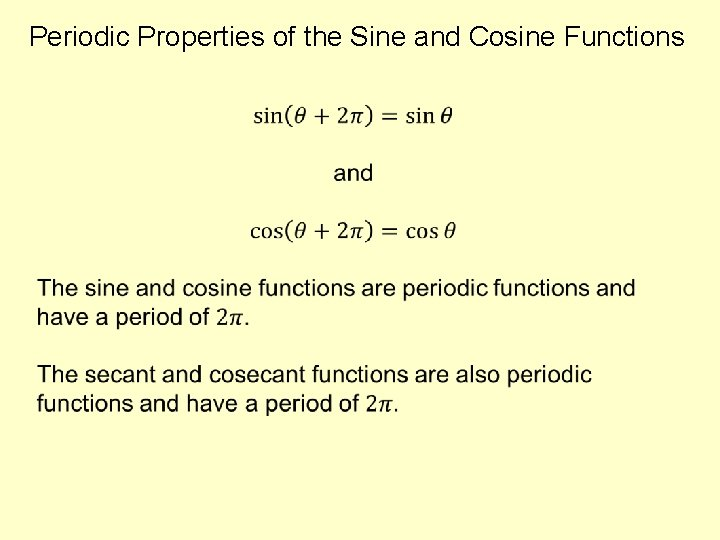Periodic Properties of the Sine and Cosine Functions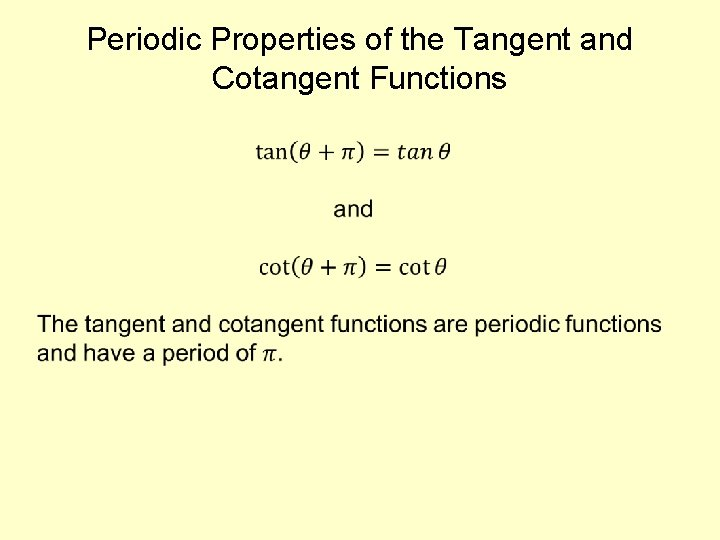Periodic Properties of the Tangent and Cotangent FunctionsSine and cosine are periodic with a period of 360 or 2 . Let's label the unit circle with values of the tangent. (Remember this is just y/x) We see that they repeat every so the tangent’s period is .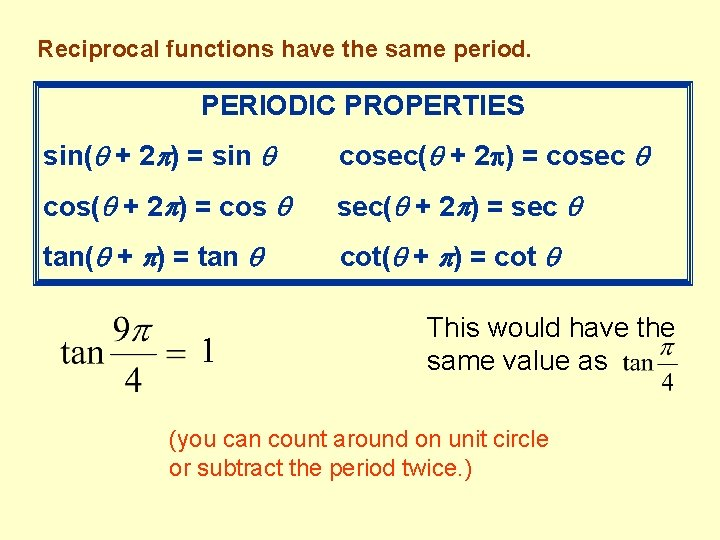Reciprocal functions have the same period. PERIODIC PROPERTIES sin( + 2 ) = sin cosec( + 2 ) = cosec cos( + 2 ) = cos sec( + 2 ) = sec tan( + ) = tan cot( + ) = cot 1 This would have the same value as (you can count around on unit circle or subtract the period twice. )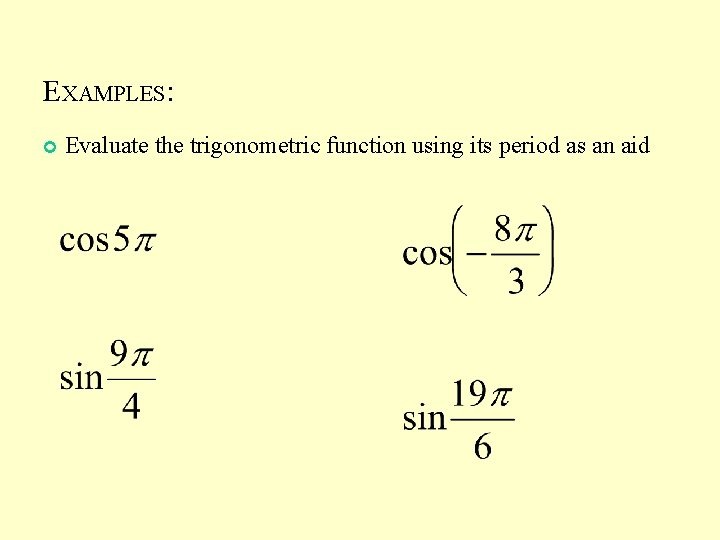EXAMPLES: Evaluate the trigonometric function using its period as an aidUsing A Calculator to Evaluate Trigonometric Functions Refer to pages 485 – 486 Go to mode Set to radians